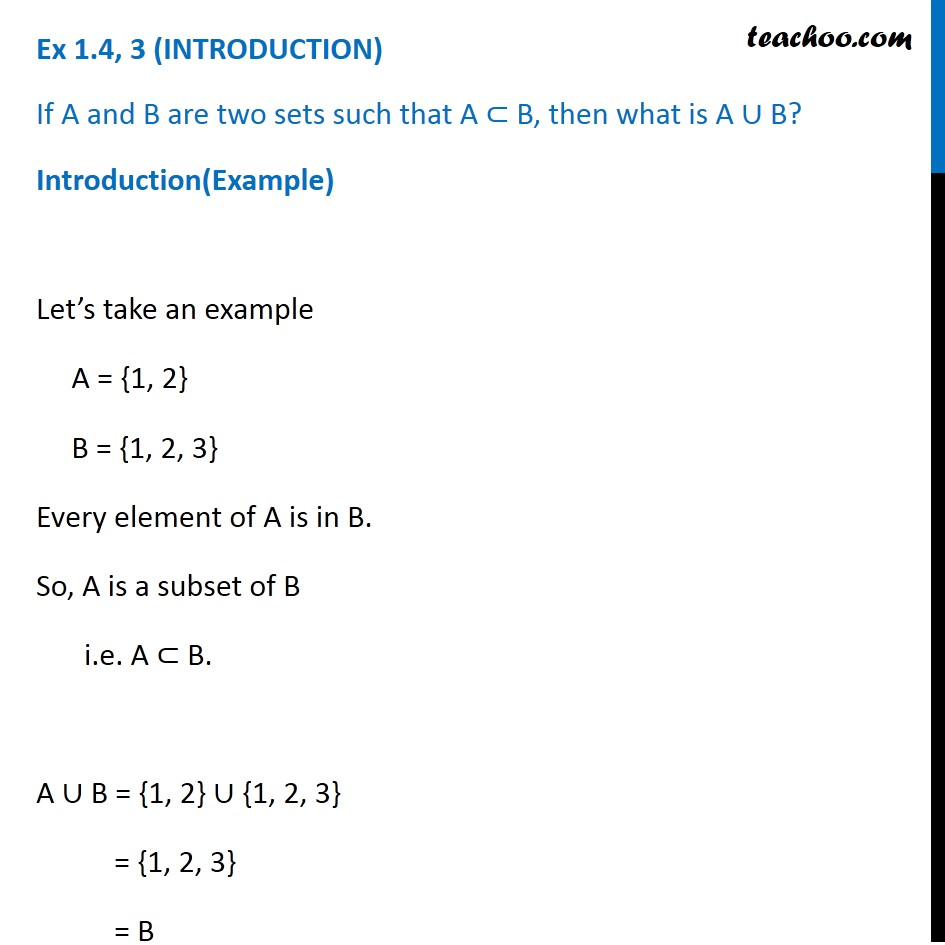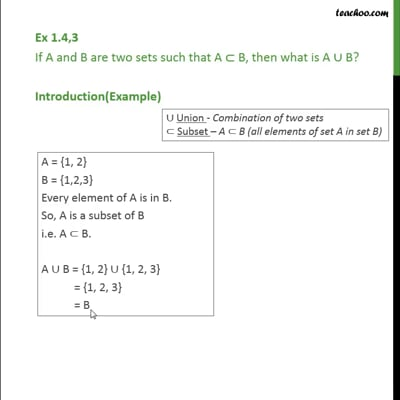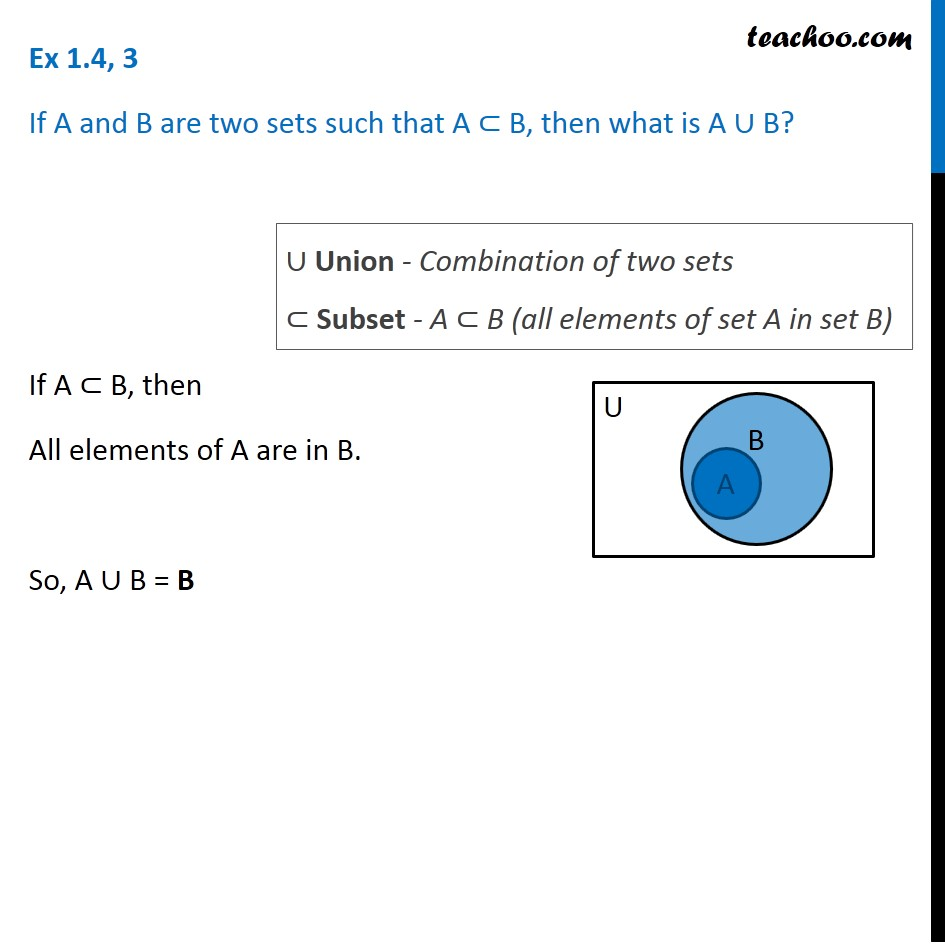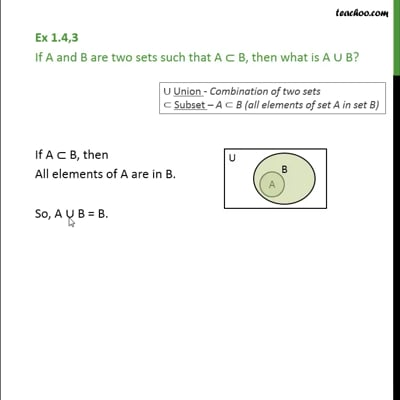Ex 1.4

Chapter 1 Class 11 Sets
Serial order wiseThis video is only available for Teachoo black usersThis video is only available for Teachoo black users

Solve all your doubts with Teachoo Black (new monthly pack available now!)

### Transcript

Ex 1.4, 3 (INTRODUCTION) If A and B are two sets such that A ⊂ B, then what is A ∪ B? Introduction(Example) Let’s take an example A = {1, 2} B = {1, 2, 3} Every element of A is in B. So, A is a subset of B i.e. A ⊂ B. A ∪ B = {1, 2} ∪ {1, 2, 3} = {1, 2, 3} = B Ex 1.4, 3 If A and B are two sets such that A ⊂ B, then what is A ∪ B? If A ⊂ B, then All elements of A are in B. So, A ∪ B = B ∪ Union - Combination of two sets ⊂ Subset - A ⊂ B (all elements of set A in set B)